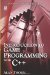# 10.5 Overloading Operators

## 10.5 Overloading Operators

In C++, two integers can be added together simply by using the plus operator (+), subtracted using the minus operator (–), and so on. But what is the sum of adding two Frogs? The following code could be written, but it will not compile since we have not defined how the two Frog classes should be added:

`      Frog frog1;      Frog frog2;      Frog frog3 = frog1 + frog2;     //Currently is not valid `

The programmer must first determine which properties of Frog must be added. Should we add all the properties of each Frog together, like Color, Width, and Height, or just one property? In C++, a programmer can establish the rules for how two classes must respond when combined using operators such as +, , *, /, and so on. The process of doing this is called overloading operators.

### 10.5.1 Overloading Operators in Practice

Overloading an operator for a class means defining how the class responds when an operator is applied to it. This definition of the operator's behavior is written in a method, the result of which returns the actual result of the operator. If the method accepts an argument, the argument will be the class that is combined with the operator. Consider the following code to define a + and operator for the Frog class.

`      #include <iostream>      class Frog      {         int Color;         int Height;         int Width;      public:         void Jump();         void Sleep();         void Eat();         int operator+(Frog param);    //Defines Add Operator         int operator-(Frog param);    //Defines Add Operator      };      void Frog::Jump()      {         //do something here      }      void Frog::Sleep()      {         //do something here      }      void Frog::Eat()      {         //do something here      }      int Frog::operator+(Frog param)      {         return Color + param.Color + Height + param.Height + Width + param.Width;      }      int Frog::operator-(Frog param)      {         return Color - param.Color - Height - param.Height - Width - param.Width;      }      int main()      {         Frog Frg1;         Frog Frg2;         int Result = Frg1 + Frg2;            return 0;      } `

 Note Notice how the keyword operator prefixes the actual operator to be overloaded; for example, operator+. This defines how behavior is handled when used in combination with this class, such as in the statement object1 + object2. Here, the parameter for the operator+ function is the second operand (object2).Introduction to Game Programming with C++ (Wordware Game Developers Library)
ISBN: 1598220322
EAN: 2147483647
Year: 2007
Pages: 225
Authors: Alan Thorn

Similar book on Amazon

flylib.com © 2008-2017.
If you may any questions please contact us: flylib@qtcs.net